# insertParkingSpaces

Insert parking spaces into parking lot

## Syntax

``insertParkingSpaces(lot,space,numSpaces,Position=position)``
``insertParkingSpaces(___,Name=Value)``
``insertParkingSpaces(lot,space,Edge=edge)``
``insertParkingSpaces(lot,space,numSpaces,Edge=edge)``
``insertParkingSpaces(___,Edge=edge,Name=Value)``

## Description

The `insertParkingSpaces` function inserts a grid of parking spaces into a parking lot at a specified position or along specified edges.

• To insert spaces at a specified position, use the `Position` name-value argument.

• To insert spaces along specified edges, use the `Edge` name-value argument.

To use this function, you must specify either `Position` or `Edge`, but you cannot specify both.

### Insert Spaces at Specified Position

````insertParkingSpaces(lot,space,numSpaces,Position=position)` inserts a one-row parking grid containing `numSpaces` spaces of type `space` into a parking lot, `lot`. The function inserts the grid at the specified xy-`position` in meters. ```

example

````insertParkingSpaces(___,Name=Value)` sets options using name-value arguments, in addition to the input arguments from the previous syntax. You can specify `Position` and the other name-value arguments in any order after the other input arguments.Example: `insertParkingSpaces(lot,space,8,Rows=2,Position=[25 25])` inserts a 2-by-8 grid of parking spaces into a parking lot at position (25, 25) in meters from the scenario origin.```

### Insert Spaces Along Specified Edges

example

````insertParkingSpaces(lot,space,Edge=edge)` inserts a one-row grid of spaces of type `space` along the specified edges `edge` of a parking lot, `lot`. The function inserts as many spaces as fit the edges.```

example

````insertParkingSpaces(lot,space,numSpaces,Edge=edge)` inserts `numSpaces` spaces along each specified edge.```

example

````insertParkingSpaces(___,Edge=edge,Name=Value)` sets options using name-value arguments, in addition to any combination of input arguments from previous syntaxes. You can specify `Edge` and the other name-value arguments in any order after the other input arguments.Example: `insertParkingSpaces(lot,space,Edge=[1,3],Offset=5)` inserts a row of parking spaces along edges 1 and 3 of a parking lot and offsets each row from its edge by 5 meters.```

## Examples

collapse all

Create a driving scenario containing a short road that enters into a parking lot that is 50 meters long and 20 meters wide. Plot the parking lot and display the edge numbers along which you can insert parking spaces. Because edge 3 forms a junction with the road, its edge number does not display on the plot, but you can still insert spaces along it.

```scenario = drivingScenario; roadcenters = [25 30; 25 15]; road(scenario,roadcenters); vertices = [0 0; 50 0; 50 20; 0 20]; lot = parkingLot(scenario,vertices); plot(scenario,ParkingLotEdges="On")```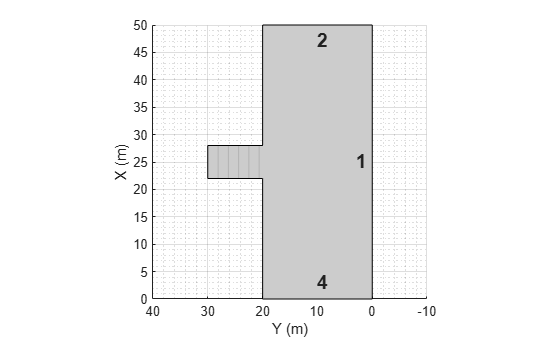Define the parking space type to add along one of the edges. Set an angle of 60 degrees. Plot the parking space.

```space = parkingSpace(Angle=60); plot(space)```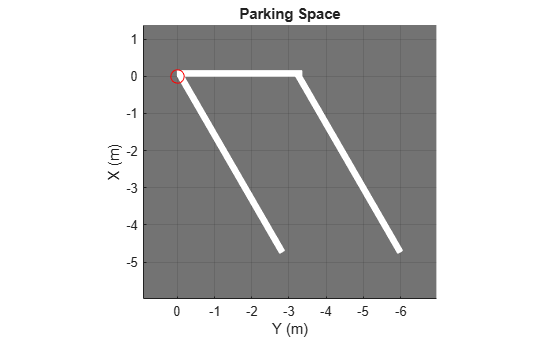Insert the angled spaces along edge 1 of the parking lot.

`insertParkingSpaces(lot,space,Edge=1)`Insert angled spaces along edge 3: five above the junction and five below the junction. Offset these spaces by 3 meters and 30 meters from the bottom of edge 3, respectively. Reverse the angle of the spaces used along edge 1.

```numSpaces = 5; space = parkingSpace(Angle=120); insertParkingSpaces(lot,space,numSpaces,Edge=3,Offset=3) insertParkingSpaces(lot,space,numSpaces,Edge=3,Offset=30)```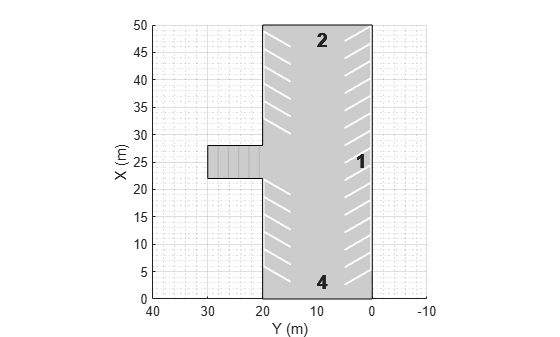Create a driving scenario containing a 50-meter road and a 20-by-30 meter parking lot. Plot the scenario.

```scenario = drivingScenario; roadcenters = [0 50; 50 0]; road(scenario,roadcenters); vertices = [40 10; 25 -5; 5 15; 20 30]; lot = parkingLot(scenario,vertices); plot(scenario)```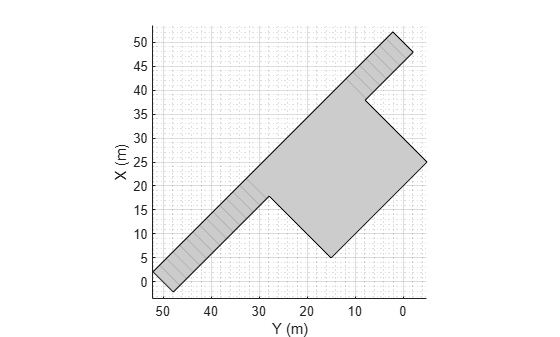Insert a parking grid into the lot. Specify a grid with two rows of eight spaces. Use the default parking space dimensions, and place the grid at a 45-degree angle to align it with the road.

```space = parkingSpace; numSpaces = 8; insertParkingSpaces(lot,space,numSpaces,Rows=2,Position=[15 20],Orientation=45)```Create a parking lot that contains a mixture of parking spaces, no-parking areas, and accessible spaces.

Define the parking space to use in the parking lot. Use the default settings. Plot the space.

```space = parkingSpace; plot(space,Origin="off")```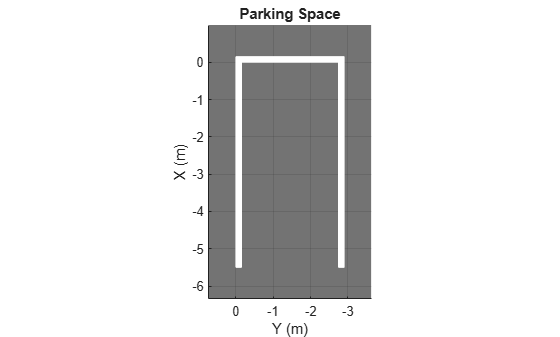Define the no-parking areas to use in the parking lot. Specify a color of white and a width that is one meter less than the width of the default parking space. Plot the space.

```noSpace = parkingSpace(Type="NoParking",Width=(space.Width - 1),MarkingColor="White"); plot(noSpace,Origin="off")```Define the accessible parking space to use in the parking lot. Specify a width that is one meter more than the width of the default parking space. Plot the space.

```accessibleSpace = parkingSpace(Type="Accessible",Width=(space.Width + 1)); plot(accessibleSpace,Origin="off")```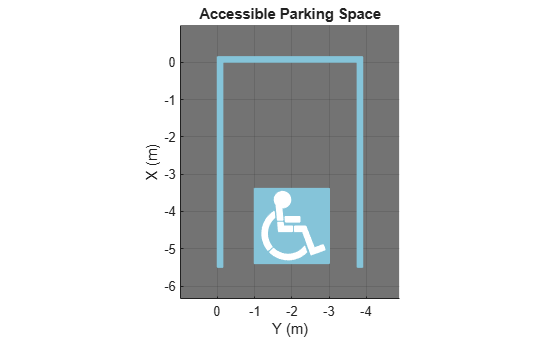Create a driving scenario containing a parking lot with a nonrectangular layout. Plot the parking lot and display the edge numbers along which you can add parking spaces.

```scenario = drivingScenario; vertices = [0 0; 32 -10.5; 32 -50.5; 25 -57.5; 0 -57.5]; lot = parkingLot(scenario,vertices); plot(scenario,ParkingLotEdges="on")```Insert default parking spaces along the first three edges of the parking lot. To avoid overlapping parking spaces, make these adjustments to the insertions:

• Along edge 1, insert only 10 spaces.

• Along edge 2, offset the spaces by 5 meters from the first vertex of the edge.

• Along edge 3, offset the spaces by 3 meters from the first vertex of the edge.

```numSpaces = 10; insertParkingSpaces(lot,space,numSpaces,Edge=1) insertParkingSpaces(lot,space,Edge=2,Offset=5) insertParkingSpaces(lot,space,Edge=3,Offset=3)```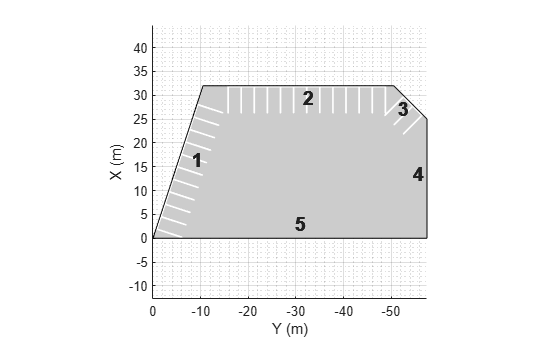In the center of the parking lot, insert a 2-by-10 grid of parking spaces containing 8 columns of default spaces, 1 column of no-parking areas, and 1 column of accessible spaces.

`insertParkingSpaces(lot,[space noSpace accessibleSpace],[8 1 1],Position=[12 -18],Rows=2)`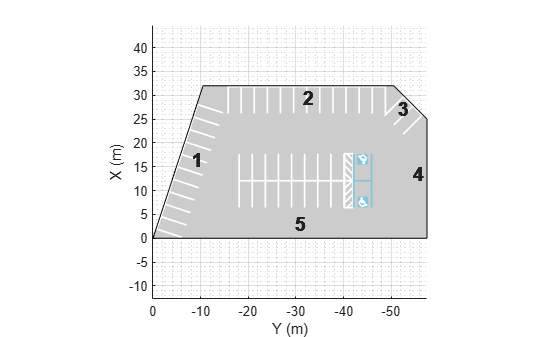## Input Arguments

collapse all

Parking lot in which to insert spaces, specified as a `ParkingLot` object. To create a parking lot, use the `parkingLot` function.

Parking spaces to insert, specified as a `parkingSpace` object or vector of `parkingSpace` objects.

`space` and `numSpaces` must contain the same number of elements. Each element of `numSpaces` specifies the number of parking spaces, of the type specified in the corresponding position of `space`, to include in the parking grid.

Example: ```[parkingSpace parkingSpace(Type="NoParking") parkingSpace(Type="Accessible")]```

Number of spaces in the parking grid, specified as a positive integer or vector of positive integers.

`space` and `numSpaces` must contain the same number of elements. Each element of `numSpaces` specifies the number of parking spaces, of the type specified in the corresponding position of `space`, to include in the parking grid.

The inserted parking grid contains `sum(numSpaces)` parking spaces. If you specify more spaces than can fit the parking lot, then the inserted parking spaces extend past the parking lot border.

Example: `[5 1 1]`

Position at which to insert the parking grid, specified as a row vector of the form `[x y]` in meters from the scenario origin. The `insertParkingSpaces` function determines the elevation (z-value) of the parking grid based on the elevation of the input parking lot `lot`.

• For one-row parking grids (`Rows=1`), `position` specifies the upper-left corner of the parking grid.

• For two-row parking grids (`Rows=2`), `position` specifies the midpoint of the left-most parking lane marking.

This table shows sample positions for one-row and two-row parking grids.

Position (One-Row Parking Grid)Position (Two-Row Parking Grid)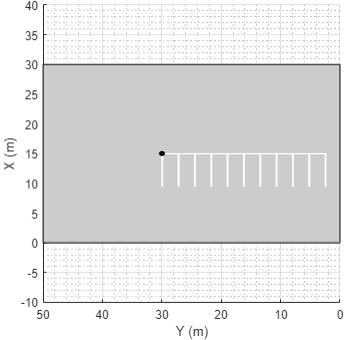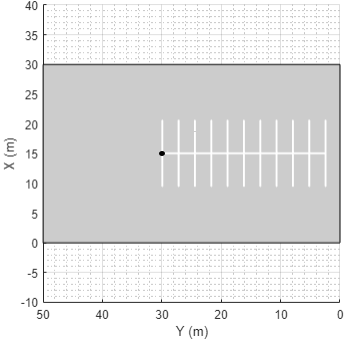Example: `[10 15]`

Edges along which to insert a parking grid, specified as a positive integer or vector of positive integers. Valid edge values are in the range [1, M], where M is the maximum number of edges in the parking lot. To view the edges along which you can insert a parking grid, use the `plot` function to plot the driving scenario that contains the parking lot, `lot`, and turn parking lot edges on. Sample code:

`plot(scenario,ParkingLotEdges="on")`

Example: `[2 4]`

### Name-Value Arguments

Specify optional pairs of arguments as `Name1=Value1,...,NameN=ValueN`, where `Name` is the argument name and `Value` is the corresponding value. Name-value arguments must appear after other arguments, but the order of the pairs does not matter.

Example: ```insertParkingSpaces(lot,space,Orientation=45,Position=[50 35])``` inserts a parking grid at a 45-degree angle at position (50, 35) in meters from the scenario origin.

Number of rows in the parking grid, specified as `1` or `2`.

#### Dependencies

To use this argument, you must specify the `position` argument.

Orientation of the parking grid, in degrees, specified as a nonnegative real scalar. Orientation is measured counterclockwise with respect to the horizontal axis of the parking grid.

• For one-row parking grids (`Rows=1`), the horizontal axis is the top edge of the grid.

• For two-row parking grids (`Rows=2`), the horizontal axis is the center line of the grid.

The table shows sample orientations for one-row and two-row parking grids.

Orientation (One-Row Parking Grid)Orientation (Two-Row Parking Grid)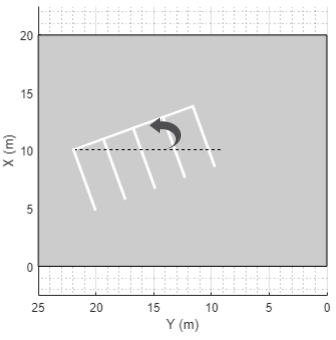#### Dependencies

To use this argument, you must specify the `position` argument.

Offset of the parking grid from the edges, in meters, specified as a nonnegative real scalar. `Offset` is relative to the first vertex of each specified edge. The same offset applies to all edges.

#### Dependencies

To use this argument, you must specify the `edge` argument.

## Version History

Introduced in R2021b